## Intro

Recently we ran sims using more realistic Pole and Chile hit maps which can be seen here. The final $$\sigma(r)$$ results can be seen in Figure 2 here. In this post I try to understand the form of that plot.

## Summary

At left are the (lensed-LCDM) signal and noise levels in the $$\ell=80$$ BB bandpower at 95GHz for the different masks. We see that circular and Pole masks are signal dominated down to low $$A_L$$. Taking these levels and running a toy sim (see below) I get relative bandpower uncertainties shown at right - to be compared to fig2 from Ben's post. Mostly the behavior is reproduced but the Pole mask does relatively a bit better here than in the full sims, and this needs further investigation.

Fig0: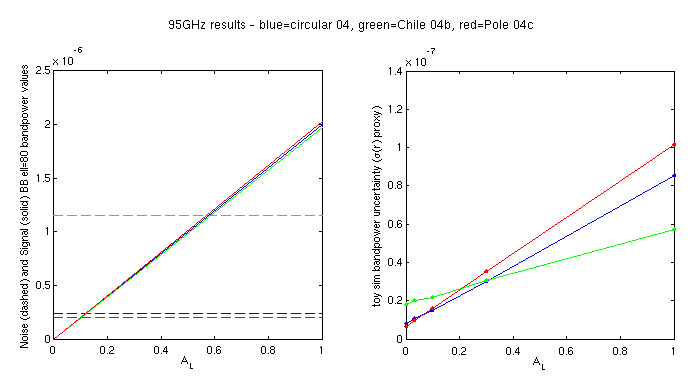[The assumption being made above is that $$\sigma(r)$$ is proportional to the bandpower uncertainty in the central frequency bands. Here by signal we mean lensed-LCDM signal since effectively the foregrounds "go away" in the cross correlation analysis. For the signal dominated cases above it would of course be possible to reduce $$\sigma(r)$$ by diverging from the standard noise optimized weighting.]

## Toy sim setup

As one spreads hits over the sky in an increasingly non-uniform manner the effective $$f_{sky}$$ for signal and noise diverge (for a given mask). Below I run some toy models to probe this effect ignoring details like E/B separation. Perhaps someone can derive the results analytically - in fact it looks like Ben basically already has here?

Let's assume there is a vector of sky signal values in pixels $$\mathbf{s}$$ - for simplicity we will let them have unit variance. Let's further assume the sky has been observed with two hit maps $$\mathbf{h_1}$$ and $$\mathbf{h_2}$$ where these both have the same sum - i.e. equal experimental effort has been expended on each: \begin{eqnarray} \sum \mathbf{h_1}=\sum \mathbf{h_2} \end{eqnarray}

For simplicity we set the peak of both hit maps to unity. The first will be a simple binary circle and the second a $$\cos^2$$ taper.

The noise will blow up around the edge of the map due to reduced observation weight there according to \begin{eqnarray} \mathbf{n}_1 = \frac{\mathbf{n}}{\sqrt{\mathbf{h}_1}} \\ \mathbf{n}_2 = \frac{\mathbf{n}}{\sqrt{\mathbf{h}_2}} \\ \end{eqnarray} (where of course it actually remains uniform for the first mask). Here we will take $$\mathbf{n}$$ as unit variance also such that signal and noise have equal fluctuation in the center of the map. (Note that this is not what we normally mean when we say signal and noise have equal power - normally we mean they have equal power post masking.)

We normally multiply the map by a mask, take the Fourier (or Spherical harmonic transform), square, and average in an annulus (or over some range in $$\ell$$) to get a "bandpower". The bandpower is thus the variance of Fourier modes. Customarily the mask is (basically) just the hit map - the inverse variance map of the noise. Here I will simply take the variance of the map times mask: \begin{eqnarray} V_{\mathbf{s}_1}' = \langle (\mathbf{s} \mathbf{h}_1)^2 \rangle \\ V_{\mathbf{s}_2}' = \langle (\mathbf{s} \mathbf{h}_2)^2 \rangle \\ V_{\mathbf{n}_1}' = \langle (\mathbf{n}_1 \mathbf{h}_1)^2 \rangle \\ V_{\mathbf{n}_2}' = \langle (\mathbf{n}_2 \mathbf{h}_2)^2 \rangle \end{eqnarray}

We require the estimate of the variance of the signal map to remain unchanged by the application of the mask - we want to measure the absolute fluctuation of the signal sky. We therefore apply a scale factor to undo the effect of the mask. This scale factor is the mean of the mask values squared: \begin{eqnarray} V_{\mathbf{s}_1} = V_{\mathbf{s}_1}' \langle \mathbf{h}_1^2 \rangle \\ V_{\mathbf{s}_2} = V_{\mathbf{s}_2}' \langle \mathbf{h}_2^2 \rangle \\ V_{\mathbf{n}_1} = V_{\mathbf{n}_1}' \langle \mathbf{h}_1^2 \rangle \\ V_{\mathbf{n}_2} = V_{\mathbf{n}_2}' \langle \mathbf{h}_2^2 \rangle \end{eqnarray}

The above two steps together are just a weighted variance. I write it as two steps since that is the way it is normally implemented when the FT/SHT is being done as an intermediate step.

In the plot below we show the signal $$\mathbf{s}$$, the hit maps $$\mathbf{h}_1$$ and $$\mathbf{h}_2$$ and the resulting instrumental noise maps $$\mathbf{n}_1$$ and $$\mathbf{n}_2$$:

Fig1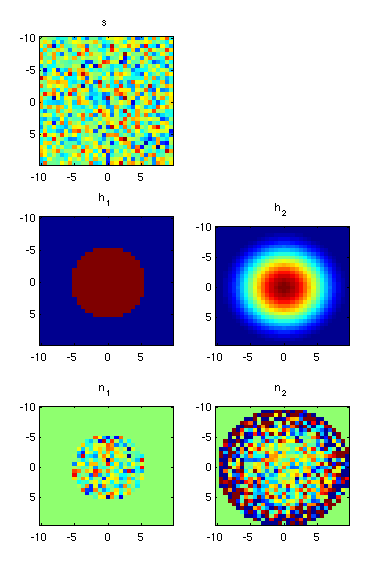In the plot below we take the product of the signal and noise maps with the masks. Note that in case 2 since the noise is rising up towards the edge it partially "resists" the apodization and covers a larger region of sky than the signal - hence it has more modes.

Fig2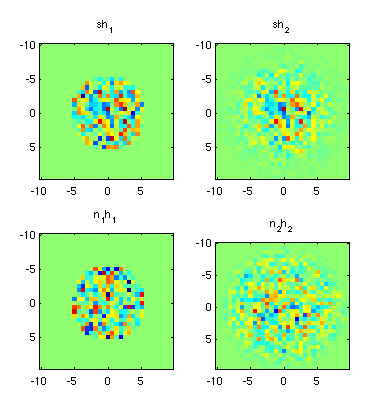Generating $$10^5$$ realizations of the above and histogramming the values gives the plot below. In the title of each panel we see the mean, standard deviation and ratio thereof for each distribution. In the upper panels we see that the signal mean value is recovered (by construction) for both masks. In the left upper and lower panels we see the expected result that for the uniform mask signal and noise behave identically. In the upper right we see that the tapered mask produces a slightly lower $$\sigma$$ value - this appears to be real and not just a fluctuation. The most important panel is the lower right. The mean noise level is 73% higher while the standard deviation is 0.12/0.089=35% higher. However, the fractional fluctuation is 1-0.070/0.089=21% lower due to the increased effective sky coverage noted above.

Fig3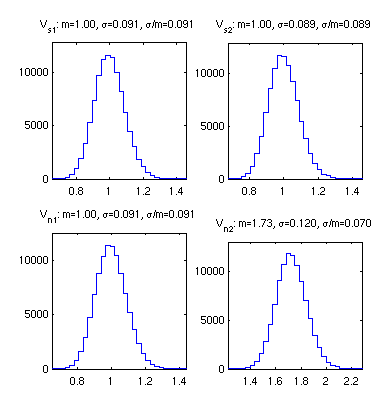In the small signal limit ($$A_L$$ small) presumably $$\sigma(N_\ell)$$ maps directly to $$\sigma(r)$$ so the above shows that the tapered mask is worse than the compact by factor 0.120/0.091 which is 32%.

### Do it on the actual healpix hit maps in question

Now let's do the above again using the hit maps in question as shown at this link. In this case they all have equal sum but only the circular one has unit peak value.

I downgrade to nside=64 to speed things up and get the below:

Fig4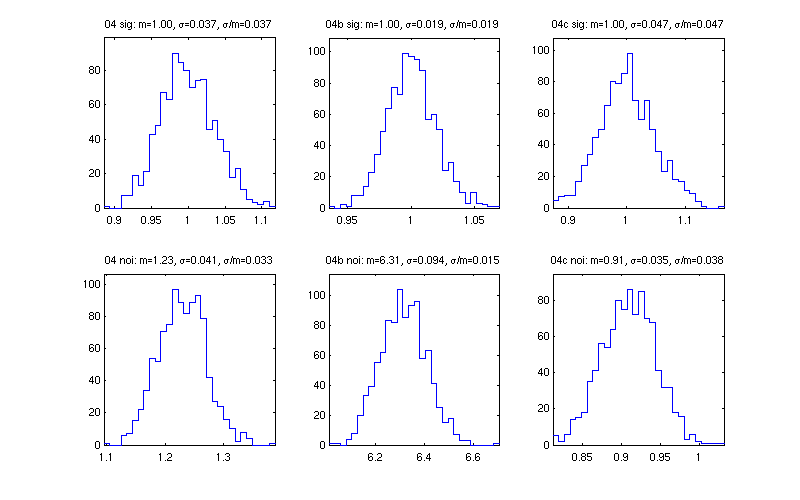The bottom row is telling us to expect slightly better performance from the 04c (Pole) hit map than from the 04 (circular) in the noise dominated regime. The ratios of the $$\sigma(N_\ell)$$ should be the same as for $$A_L=0$$ in Fig0 above.

Putting in the levels of signal and noise from Fig0 left I then run for varying levels of $$A_L$$ and produce the plot in Fig0 right.

### Strange behavior in $$N_\ell$$ Pole mask?

In this plot we see odd behavior in the 04c (Pole) $$N_\ell$$ $$EE$$ and $$BB$$ curves at low $$\ell$$ where they cross over with the 04 curves. It's not clear that the pure-B estimator could do that - maybe enhanced $$\sigma(N_\ell)$$ for diffuse mask but how to enhance $$N_\ell$$? The plot below zooms in a bit and also looks at signal to noise ratio. We expect the noise curves to kick up at low $$\ell$$ since we put in $$1/f$$ - but they should all kick up the same on a log plot. Instead blue kicks up more than green and red kicks up enough to cross over blue.

Fig5From Fig0 above it does look like perhaps the strange behavior in the plot above is effecting the Pole mask results a bit.

### Code

The code for figures 1-5 is here.

The code for figure 0 is here.